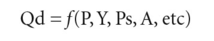# demand function

## demand function

a form of notation that links the DEPENDENT VARIABLE, quantity demanded (Qd), with various INDEPENDENT VARIABLES that determine quantity demanded such as product price (P), income (Y), prices of substitute products (Ps), advertising (A), etc.:Changes in any of these independent variables will affect quantity demanded, and if we wish to investigate the particular effect of any one of these variables upon quantity demanded, then we could (conceptually) hold the influence of the other independent variables constant (CETERIS PARIBUS), whilst we focus upon the particular effects of that independent variable. See DEMAND CURVE, DEMAND CURVE ( SHIFT IN).

Collins Dictionary of Economics, 4th ed. © C. Pass, B. Lowes, L. Davies 2005
References in periodicals archive ?
where D is the demand function, S is the supply function, and t is the cost increase or tax that all the firms in the markets incur.
The independent variables for the money demand function include a real income, exchange rate and measure of the interest rate.
However, researchers have not undertaken theoretically estimates of the demand function of the Korean ICT construction business.
The demand function in the insurance market is assumed to be linear and is specified as
DISSERTATION back in 1979, my research topic was the money demand function and the effect financial innovations had as a result.
They first modeled a demand function for products and then maximized store profit by selecting products from assortment and allocating optimally the chosen products.
This study attempts to estimate the electricity demand function for Pakistan using smooth transition autoregressive model over the period 1971-2012.
The following equations express the deriving of a demand function for the good 1 from two relations:
The results show that in Germany inflation and long-term interest rates are super-exogenous with respect to the parameters of a demand function for M3.
This uncertainty maybe concerned with information on the exact demand function, rivals' costs, or even components of firms own production cost.
Traditionally, stock market prices have not been considered as a determinant of the money demand function until Friedman (1988) showed its relevance.
money demand function using both nominal and real M1 and M2 monetary aggregates.

Site: Follow: Share:
Open / Close